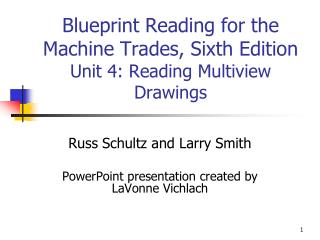Download Presentation# Blueprint Reading for the Machine Trades, Sixth Edition Unit 4: Reading Multiview Drawings - PowerPoint PPT Presentation

Blueprint Reading for the Machine Trades, Sixth Edition Unit 4: Reading Multiview Drawings. Russ Schultz and Larry Smith PowerPoint presentation created by LaVonne Vichlach. Reading the Drawings. Sufficient number of dimensions on a print to enable you to produce the drawingI am the owner, or an agent authorized to act on behalf of the owner, of the copyrighted work described.
Download PresentationDownload Policy: Content on the Website is provided to you AS IS for your information and personal use and may not be sold / licensed / shared on other websites without getting consent from its author.While downloading, if for some reason you are not able to download a presentation, the publisher may have deleted the file from their server.

- - - - - - - - - - - - - - - - - - - - - - - - - - E N D - - - - - - - - - - - - - - - - - - - - - - - - - -
Presentation Transcript
1. Blueprint Reading for the Machine Trades, Sixth Edition Unit 4: Reading Multiview Drawings Russ Schultz and Larry Smith PowerPoint presentation created by LaVonne Vichlach

2. Reading the Drawings • Sufficient number of dimensions on a print to enable you to produce the drawing • Each dimension appears only once • Drafter may place dimension on different view than expected

3. Reading the Drawings • Need to be proficient at reading multiview drawings • May need to calculate dimensions not provided from surrounding information

4. Spacer Block Calculate A .62

5. Spacer Block Calculate B .66

6. Spacer Block Calculate C .64

7. Spacer Block Calculate D 1.94

8. Spacer Block Calculate E 1.04

9. Spacer Block Calculate F 1.28

10. Spacer Block Calculate G 1.30

11. Spacer Block Calculate H 1.92

12. Spacer Block Calculate I 1.14

13. Spacer Block Calculate J .10

14. Spacer Block Calculate K 1.94

15. Spacer Block Calculate L .82

16. Spacer Block Calculate M 1.04

17. Basic Dimension Quiz Which of the three basic dimensions are shown in the front view-height, width, or depth? Height Width

18. Basic Dimension Quiz Which of the three basic dimensions are shown in the top view-height, width, or depth? Width Depth

19. Basic Dimension Quiz Which of the three basic dimensions are shown in the right-side view-height, width, or depth? Height Depth

20. Basic Dimension Quiz Which of the three basic dimensions are shown in the bottom view-height, width, or depth? Width Depth

21. Basic Dimension Quiz Which of the three basic dimensions are shown in the left-side view-height, width, or depth? Height Depth

22. Basic Dimension Quiz Which of the three basic dimensions are shown in the rear view-height, width, or depth? Height Width

23. Dimension Calculations 1 1. Height dimension? 1.30

24. Dimension Calculations 1 2. Width dimension? 2.00

25. Dimension Calculations 1 3. Depth dimension? 1.10

26. Dimension Calculations 1 Calculate A .50

27. Dimension Calculations 1 Calculate B .80

28. Dimension Calculations 1 Calculate C .70

29. Dimension Calculations 1 Calculate D .50

30. Dimension Calculations 1 Calculate E .60

31. Dimension Calculations 1 Calculate F .20

32. Dimension Calculations 1 Calculate G 1.30

33. Dimension Calculations 1 Calculate H 2.00

34. Dimension Calculations 1 Calculate I 1.50

35. Dimension Calculations 1 Calculate J .50

36. Dimension Calculations 1 Calculate K .60

37. Dimension Calculations 1 Calculate L 1.10

38. Dimension Calculations 1 Calculate M .60

39. Dimension Calculations 1 Calculate N .50

40. Precedence of Lines When visible lines, hidden lines, or centerlines coincide in same view-a preferred line is illustrated.

41. Precedence of Lines • Visible line over hidden line • Visible line over centerline • Hidden line over centerline

42. Precedence of Lines

43. ADJ Holding Block 1. What is the name of the object? Adj Holding Block

44. ADJ Holding Block 2. Of what material is the object made? (Do not abbreviate.) Hot-rolled steel

45. ADJ Holding Block 3. To what scale (half, full, double) is the object drawn? Full

46. ADJ Holding Block 4. What system of dimensioning (fractional, decimal, metric) is used? Metric

47. ADJ Holding Block 5. What decimal increments (inches, centimeters, millimeters) are used? Millimeter

48. ADJ Holding Block 6. What angle of projection (first, second, third) is used? Third

49. ADJ Holding Block 7. What tolerance is assigned to each dimension? 0.5 MM

50. ADJ Holding Block 8. What is the height dimension? 38 MM